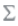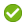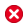## Creating Formulas

When you select a cell, column, or row containing a formula, you can apply a unique formula to that cell. When you create formulas, keep in mind the following points:

• For optimal performance, use row or column formulas as opposed to cell formulas whenever possible.

• Avoid using cross-axis references and consider using a cell reference if possible.

To create a formula:

1. In a grid, insert a formula row or column and select the formula cell.

To apply a formula to the entire column or row, click the column or row header cell.

When you use a cell formula in a row or column formula, the cell formula should reference the cells exactly (intersection) and not just the row and column.

2. Do one of the following:
• Enter a formula manually.

• Click, select a function from the drop-down list, and enter the formula parameters.

See Using Functions.

3. Clickto validate the formula, or clickto clear the formula bar and start over.